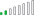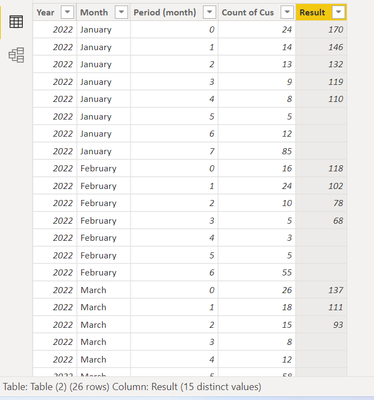cancel
Showing results for
Did you mean:Helper I

## HELP!!! Reversed cumulative total by group with criteria

Hi, I am working on building a customer retention analysis.

Below is my sample data- New customer counts by year month.

Column "Periods" is a calculated column, breaking down those customers who joined at that year-month, and how many months they have been with us.

 Year Month Period (month) Count of Cus 2022 January 0 24 170 2022 January 1 14 146 2022 January 2 13 132 2022 January 3 9 119 2022 January 4 8 110 2022 January 5 5 2022 January 6 12 2022 January 7 85 2022 February 0 16 118 2022 February 1 24 102 2022 February 2 10 78 2022 February 3 5 68 2022 February 4 3 2022 February 5 5 2022 February 6 55 2022 March 0 26 137 2022 March 1 18 111 2022 March 2 15 93 2022 March 3 8 2022 March 4 12 2022 March 5 58 2022 April 0 18 145 2022 April 1 25 127 2022 April 2 13 2022 April 3 11 2022 April 4 78My main struggles are 2 points:

1. I would like to calculate the Reversed Running Total by each month as a group (decreasing by periods)

2. Due to the business logic, I would like to omit the latest 3 periods of each month group.

The preferred result is the most right column. And since most of the columns in my data are calculated columns, solutions via power query will not be ideal. Thank you in advance!

1 ACCEPTED SOLUTIONResolver II

Hi @jts_

You can add a calculated column with this code.

``````Result =
VAR _period = 'Table (2)'[Period (month)]
VAR _latestPeriod = CALCULATE( MAX('Table (2)'[Period (month)]), ALLEXCEPT('Table (2)', 'Table (2)'[Year], 'Table (2)'[Month]))
RETURN
IF( _period < _latestPeriod - 2, CALCULATE( SUM('Table (2)'[Count of Cus]), ALLEXCEPT('Table (2)', 'Table (2)'[Year], 'Table (2)'[Month]), 'Table (2)'[Period (month)] >= _period), BLANK())``````Cheers

If this reply helps solve the problem, please mark it as Solution! Kudos are appreciated too!

3 REPLIES 3Resolver II

Hi @jts_

You can add a calculated column with this code.

``````Result =
VAR _period = 'Table (2)'[Period (month)]
VAR _latestPeriod = CALCULATE( MAX('Table (2)'[Period (month)]), ALLEXCEPT('Table (2)', 'Table (2)'[Year], 'Table (2)'[Month]))
RETURN
IF( _period < _latestPeriod - 2, CALCULATE( SUM('Table (2)'[Count of Cus]), ALLEXCEPT('Table (2)', 'Table (2)'[Year], 'Table (2)'[Month]), 'Table (2)'[Period (month)] >= _period), BLANK())``````Cheers

If this reply helps solve the problem, please mark it as Solution! Kudos are appreciated too!Helper I

Thanks for the help! It works great!!Super User